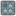• # Extra Test 3/4

## Question related to missionMoore Neighbourhood

At "Extra Test 3/4" I'm getting this error:

"IndexError: tuple index out of range, count_neighbours, 5"

My code is:

```def count_neighbours(grid, row, col):
a = 0
for x in (-1,0,1):
for y in (-1,0,1):
if 0 <= row+x < len(grid) and 0 <= col+y < len(grid) and (row, col) != (row+x,col+y) and (grid[row+x][col+y]):
a += 1
return a
```

What is so special about "Extra Test 3/4" or my code that it doesn't succeeded?

p.s. There is no error in Output (Python Console) - is it because it is "Extra" test?8